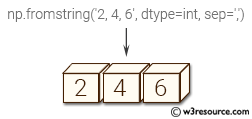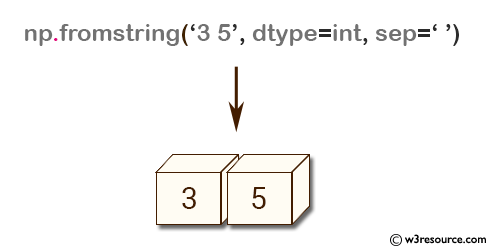# NumPy array creation: fromstring() function

## fromstring() function

The fromstring() function is used to create a new 1-D array initialized from raw binary or text data in a string.

Syntax:

`numpy.fromstring(string, dtype=float, count=-1, sep='')`Version: 1.15.0

Parameter:

Name Description Required /
Optional
string A string containing the data. Required
dtype The data type of the array; default: float. For binary input data, the data must be in exactly this format Optional
count Read this number of dtype elements from the data. If this is negative (the default), the count will be determined from the length of the data. Optional
sep The string separating numbers in the data; extra whitespace between elements is also ignored. Optional

Return value:

arr [ndarray]
The constructed array.

Raises:

ValueError If the string is not the correct size to satisfy the requested dtype and count.

Example-1: NumPy.fromstring() method

``````>>> import numpy as np
>>> np.fromstring('3 5', dtype=int, sep=' ')
array([3, 5])
``````

Pictorial Presentation:Example-2: NumPy.fromstring() method

``````>>> import numpy as np
>>> np.fromstring('3, 5', dtype=int, sep=',')
array([3, 5])
``````

Python - NumPy Code Editor:

Previous: fromiter()
Next: loadtxt()

﻿